Practice the AP 8th Class Physical Science Bits with Answers Chapter 1 Force on a regular basis so that you can attempt exams with utmost confidence.

## AP State Syllabus 8th Class Physical Science Bits 1st Lesson Force with Answers

Question 1.
The S.I. unit of force is
A) Pascal
B) Newton
C) Square metre
D) Tesla
B) Newton

Question 2.
The resisting force to movement of an object is called
A) friction
B) magnetic force
C) tension
D) normal force
A) frictionQuestion 3.
………… force acts on the suspended body along the string.
A) Friction
B) Tension
C) Magnetic force
D) Muscular force
B) Tension

Question 4.
The forces exerted by a charged body on another charged or uncharged body is known as
A) Electrostatic force
B) Friction
C) Tension
D) Normal force
A) Electrostatic force

Question 5.
When the object is in non-uniform motion, it is said to be in
A) displacement
B) momentum
C) acceleration
D) weight
C) acceleration

Question 6.
The force that a solid surface exerts on any object in the normal direction is called
A) Muscular force
B) Normal Force
C) Tension force
D) Magnetic force
B) Normal ForceQuestion 7.
Which of the following statements is correct about a force?
A) It can be seen but it can’t be felt.
B) It is needed to start an object moving.
C) It can change the shape of an object.
D) It can make moving object stop.
A) It can be seen but it can’t be felt.

Question 8.
Pressure can be increased by
A) Increasing both the area and the force.
B) Decreasing the area and increasing the force.
C) Increasing the area and decreasing the force.
D) Decreasing both the area and the force.
B) Decreasing the area and increasing the force.

Question 9.
Pressure varies with force (F) as (provided area is same)
A) F
B) $$\frac{1}{F}$$
C) F2
D) $$\frac{1}{\mathrm{~F}^{2}}$$
A) F

Question 10.
An action that involves pushing force is
A) Dragging a bag on a floor.
B) Kicking a ball.
C) Lifting a book from a table.
D) Lifting a pen off a table.
B) Kicking a ball.Question 11.
Pressure depends on and
A) Mass, Force
B) Mass, Volume
C) Force, Area
D) Force, Volume
C) Force, Area

Question 12.
Force has ………. as well as ………
A) mass, weight
B) magnitude, direction
C) mass, action
D) action, reaction
B) magnitude, direction

Question 13.
Pressure exerted by a sharp needle on a surface is
A) More than the pressure exerted by a blunt needle.
B) Less than the pressure exerted by a blunt needle.
C) Equal to the pressure exerted by a blunt needle.
D) None of these.
A) More than the pressure exerted by a blunt needle.

Question 14.
A force can change ……… of a body.
A) shape
B) direction
C) speed
D) all the above
D) all the aboveQuestion 15.
The force acting on unit area of a surface is called
A) momentum
B) density
C) pressure
D) velocity
C) pressure

Question 16.
The unit of force in S.I. system is
i) Kg ms-2
ii) Newton
iii) Pascal
iv) Kg ms-1
A) Both i and ii
B) iii and iv
C) i and iii
D) i and iv
A) Both i and ii

Question 17.
The unit of pressure in S.I. system is
A) Newton
B) Pascal
C) Ampere
D) Henry
B) Pascal

Question 18.
Pressure = …………
A) Force
B) Force x area
C) Area / force
D) Force / area
D) Force / areaQuestion 19.
The force of attraction between any two objects having mass is
A) frictional
B) muscular
C) gravitational
D) magnetic
C) gravitational

Question 20.
For the body at rest, the net force is …………
A) ma
B) not equal to zero
C) 0
D) none of these
C) 0

Question 21.
For the body at motion, the net force is
A) ma
B) o
C) not equal to zero
D) none of these
C) not equal to zero

Question 22.
If the area is less, then the value of pressure
A) less
B) more
C) does not change
D) none of these
B) moreQuestion 23.
The force acting in normal direction is
A) gravitational force
B) magnetic force
C) muscular force
D) normal force
D) normal force

Question 24.
The force exerted by using our body muscles is
A) muscular force
B) magnetic force
C) gravitational force
D) normal force
A) muscular force

Question 25.
Choose correct matching option:
Group – A — Group – B
1. Gravitational force — a) Horse pulling a cart
2. Frictional force — b) Iron nails attracted
3. Electrostatic force — c) Fruit falling from a tree
4. Magnetic force — d) Heat is generated
5. Muscular — e) Bits of paper are raised
A) 1 – c, 2 – d, 3 – e, 4 – b, 5 – a
B) 1 – a, 2 – b, 3 – c, 4 – d, 5 – e
C) 1 – e, 2 – c, 3 – d, 4 – a, 5 – b
D) 1 – e, 2 – d, 3 – a, 4 – b, 5 – c
A) 1 – c, 2 – d, 3 – e, 4 – b, 5 – a

Question 26.
Choose correct matching option:
Group – A — Group – B
1. Force responsible for attraction between two objects. — a) Weight
2. Force by which the Earth, the Moon and other massive large objects attracts another object. — b) Mass
3. Force applied by muscles of our body. — c) Newton
4. Force exerted by a surface as an object moves across it. — d) Muscular
5. The amount of substance contained in an object. — e) Magnetic
6. Units of force. — f) Gravitational
7. Force due to earth is called — g) Frictional
A) 1 – e, 2 – f, 3 – d, 4 – g, 5 – b, 6 – c, 7 – a
B) 1 – a, 2 – b, 3 – c, 4 – d, 5 – e, 6 – f, 7 – g
C) 1 – b, 2 – c, 3 – d, 4 – e, 5 – f, 6 – g, 7 – a
D) 1 – c, 2 – d, 3 – e, 4 – f, 5 – g, 6 – a, 7 – b
A) 1 – e, 2 – f, 3 – d, 4 – g, 5 – b, 6 – c, 7 – aQuestion 27.
The resistance to the movement of a body over the surface of another is
A) force
B) friction
C) workdone
D) energy
B) friction

Question 28.
The direction of friction and the direction of motion are always
A) same
B) opposite
C) A or B
D) we cannot say
B) opposite

Question 29.
Direction and magnitude are fixed for
A) normal force
B) frictional force
C) tension force
D) gravitational force due to earth
D) gravitational force due to earth

Question 30.
The force acts between a charged balloon and piece of papers
A) magnetic
B) gravitational
C) contact
D) electrostatic
D) electrostaticQuestion 31.
The gravitational force acts between
B) you and earth
C) you and moon
D) above all
D) above all

Question 32.
We can differentiate ‘contact force’ and ‘field force’ by
A) magnitude
B) direction
C) distance of contact
D) above all
C) distance of contact

Question 33.
Net force is zero, when an object is in
A) uniform motion
B) rest
C) A and B
D) uniform acceleration
C) A and B

Question 34.
Which of the following is wrong?
A) Force can change the direction of an object
B) Force can change the shape of an object
C) Force can change the speed of an object
D) Force can change the mass of an object
D) Force can change the mass of an objectQuestion 35.
Normal force is applicable only when the given force is exist.
A) gravitational
B) frictional
C) both A and B
D) none
A) gravitational

Question 36.
Match it.
a) Changes the speed of an object — i) hitting a ball projected by a bowler
b) Changes the shape of an object — ii) making a ship with a paper
c) Changes the direction of an object — iii) applying brakes for a moving car
A) a – iii, b – ii, c – i
B) a – i, b – ii, c – iii
C) a – iii, b – i, c – ii
D) a – ii, b – i, c – iii
A) a – iii, b – ii, c – i

Question 37.
Which of the following is a correct statement?
A) A car is in rest, no forces acting on it.
B) A car is in rest, net force acting on it is zero.
C) A car is in non-uniform motion, net force acting on it is zero.
D) None
B) A car is in rest, net force acting on it is zero.

Question 38.
Assertion (A): Magnetic force is a field force.
Reason (R): A magnet can attract or repel another magnet with zero magnitude.
A) A and R are true
B) A and R are false
C) A is true, but R is false
D) A is false, but R is true
C) A is true, but R is falseQuestion 39.
Take two balloons inflate them. Rub both with your hair. Bring them to closer to each other. Then they
A) repels
B) attracts
C) no change
D) we cannot say anything
A) repels

Question 40.
An apple is falling from a tree. The forces acting on it
A) drag
B) gravitational
C) normal
D) above all
D) above all

Question 41.
A free falling body can stop by using
A) gravitational force
B) frictional force
C) normal force
D) any of the given
C) normal force

Question 42.
Which of the following force is exists anywhere in the universe?
A) Gravitational force
B) Magnetic force
C) Normal force
D) Above all
A) Gravitational forceQuestion 43.
What will happen, if you apply more force on a moving body?
A) Increases its speed
B) Decreases its speed
C) A or B
D) A and B
C) A or B

Question 44.
Which one of these is not a contact force?
A) Muscular force
B) Frictional force
C) Normal force
D) Magnetic force
D) Magnetic force

Question 45.
Let the forces F1 and F2 act on the table in opposite directions, F1 > F2 , the Fnet = …………….
A) F1 – F2
B) F2 + F2
C) 0
D) 2F2 – F1
A) F1 – F2

Question 46.
Which of the following statements about force is incorrect?
A) A force can change the mass of an object.
B) A force can change the speed (or) direction.
C) A force can change the shape and size of an object.
D) Forces can stop a moving object.
A) A force can change the mass of an object.Question 47.
Which of the following statements is incorrect?
A) Friction helps us to twist an object.
B) Friction is a force that opposes motion.
C) Objects have weight because of frictional force between them and the Earth.
D) More friction is produced between rough surfaces than between smooth surfaces.
C) Objects have weight because of frictional force between them and the Earth.

Question 48.
Which of the following statements is correct about force of gravity?
A) The larger the mass, the greater is gravity.
B) Force of gravity is commonly called weight.
C) It makes the moon go around the earth.
D) The larger the weight of an object, greater is the gravity.
C) It makes the moon go around the earth.

Question 49.
What will happen if a compass placed near a bar magnet?
A) Compass needle moves by muscular force.
B) Compass needle moves by gravitational force.
C) Compass needle moves by frictional force.
D) Compass needle moves by magnetic force.
D) Compass needle moves by magnetic force.

Question 50.
What happens if a boy through a stone?
A) Some muscles will expend
B) Some muscles will contract
C) Both A and B
D) Some muscles will dislocate
C) Both A and BQuestion 51.
A book on a table is in rest. Then the magnitude of the given force is zero.
A) Normal
B) Frictional
C) Gravitational
D) None
B) Frictional

Question 52.
What will happen if thread is broken?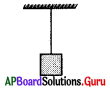A) Force of tension > Force of gravity
B) Force of friction > Force of gravity
C) Force of tension < Force of gravity
D) Force of gravity = Force of tension
C) Force of tension < Force of gravity

Question 53.
No. of forces acting on acting on the block ‘B’ is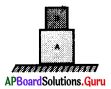A) 1
B) 2
C) 3
D) 4
B) 2Question 54.
A push or a pull is called
A) friction
B) pressure
C) force
D) none of these
C) force

Question 55.
Erasing involves
A) a push
B) a pull
C) both
D) none of these
C) both

Question 56.
Hoisting a flag is related to
A) push
B) pull
C) push and pull both
D) pressure
B) pull

Question 57.
A person is pulling water from well.
Which type of force it is?
A) Muscular force
B) Magnetic force
C) Friction force
D) Electrostatic force
A) Muscular forceQuestion 58.
With the increase in the weight of an object the pressure.
A) Increases
B) Decreases
C) Is not affected
D) None
A) Increases

Question 59.
Read and study the given actions carefully and answer correctly to “Where the friction is useful”……………
I) Striking a match stick
II) Writing with a pencil
III) Pushing a cupboard from one room to another.
IV) Sharpening a knife.
A) I and II only
B) I and III only
C) I, II and IV only
D) All of the above
C) I, II and IV only

Question 60.
In the given experiment which type of force is represented?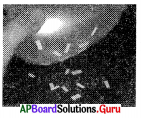A) Magnetic
B) Electrostatic
C) Gravitational
D) Tension
A) MagneticQuestion 61.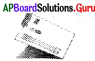From the given activity your conclusion is
a) friction depends on smoothness of the surface of an object.
b) friction depends on smoothness of the inclined plane.
A) a only
B) b only
C) a and b only
D) a or b only
C) a and b only

Question 62.
The apparatus required to find a limiting force of a thread.
A) Simple balance
B) Spring balance
C) Electronic balance
D) None
B) Spring balance

Question 63.
How do yon show to at force can change the shape of an object?
A) Squeezing a sponge with hand
B) Squeezing an iron block with hand
C) Throwing a ball
D) Stopping a ball
A) Squeezing a sponge with handQuestion 64.
From this experiment, you can conclude that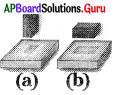A) the surface area is smaller, the pressure will be greater.
B) the surface area is greater, the pressure will be greater.
C) the surface area is smaller, the pressure remains same.
D) none
A) the surface area is smaller, the pressure will be greater.

Question 65.
From the given figure, the force which is pulling the cart is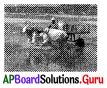A) contact force
B) force at a distance
C) muscular force
D) A and C
D) A and C

Question 66.
From the given diagram, you may noticed that F pair of forces acting in opposite directions are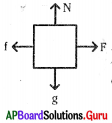a) Normal force and frictional force
b) Normal force and gravitational force
c) Frictional force and external force
d) Normal force and external force
e) Frictional force and gravitational force
A) a, b
B) b, c
C) c, d
D) d, e
B) b, cQuestion 67.
In the given diagram, field force is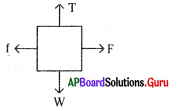A) f
B) T
C) F
D) W
D) W

Question 68.
The forces acting in the given system are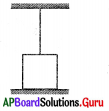A) Tension and gravitational
B) Tension and frictional
C) Tension, frictional, gravitational
D) Tension or gravitational
A) Tension and gravitational

Question 69.
Wrongly mentioned in the table is

 Force Exerts a Magnetic Around the magnet b Electrostatic Around the charge c Gravitational Around the earth

A) a
B) b
C) c
D) None
C) c

Question 70.
The force of a field is greater at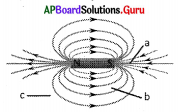A) a
B) b
C) c
D) All are equal
A) aQuestion 71.
The net force is

 Force Magnitude Direction F 40 N Towards east f 20 N Towards west T 30 N Towards up W 30 N Towards down

A) 20 N – Towards east
B) 40 N – Towards west
C) 20 N – Towards down
D) None
A) 20 N – Towards east

Question 72.
The forces acting on the body are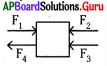A) +F1, +F2, -F3, +F4
B) -F1 + F2, -F3, +F4
C) +F1, -F2, -F3, -F4
D) +F1, -F2, -F3, +F4
C) +F1, -F2, -F3, -F4

Question 73.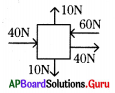The body moves in the direction of the given arrow mark
A) →
B) ←
C) ↓
D) ↑
A) →

Question 74.
Study the above diagram. Why the given object does not move?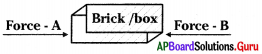A) Force – A > Force – B
B) Force – B > Force – A
C) both forces are equal.
D) both forces are acting in opposite directions.
C) both forces are equal.Question 75.
Siri wants to hit some pins in a balloon game as shown in the figure. To do so, where she should apply a force on the balloon?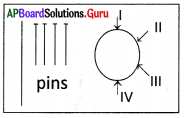A) I
B) II
C) III
D) IV
B) II

Question 76.
Study the figure given below. At which point, the pressure is greater?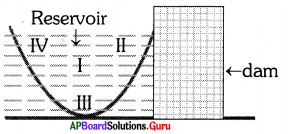A) I
B) II
C) III
D) IV
C) III

Question 77.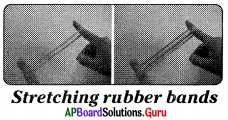From the above diagram
The force exerted by one rubber band is F. Then the net force acting on your finger when four rubber bands are used is
A) F
B) 2F
C) 3F
D) 4F
D) 4F

Question 78.
The diagram which showing all the forces acting on object at a particular instant is
A) Free body diagram
B) Free fall diagram
C) Free object diagram
D) None of these
A) Free body diagramQuestion 79.
Name the type of force you observed in the figure.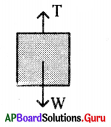A) Magnetic force
B) Gravitational force
C) Electrostatic force
D) Tension
D) Tension

Question 80.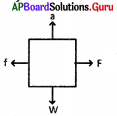The part ‘a’ is
A) S
B) N
C) P
D) G
B) N

Question 81.
The direction of force can be represented by
A) →
B) ←
C) ↑
D) Any one of the above
D) Any one of the above

Question 82.
If draw a diagram for the given data it looks like

 Force Direction A Push Towards Left B Puli Towards Right C Tension Towards Up D Gravity Towards Down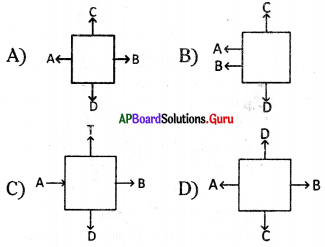A)Question 83.
The free body diagram of a moving aeroplane is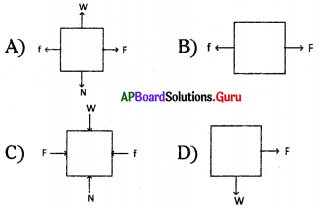C)

Question 84.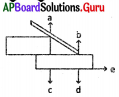Directions of normal forces labelled at
A) a, b
B) c, d
C) c
D) a, c
A) a, b

Question 85.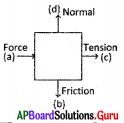The wrongly mentioned part is
A) a
B) b
C) c
D) d
B) b

Question 86.
The air which is important to us is not escaping from the Earth. This is because of
A) Normal force
B) Electrostatic force
C) Gravitational force
D) Above all
C) Gravitational force

Question 87.
This force plays great role to do work play specially for human beings.
A) Electrostatic force
B) Muscular force
C) Tension force
D) Magnetic force
B) Muscular forceQuestion 88.
Oldage people seek help because they loss their
A) muscular force
B) frictional force
C) gravitational force
D) above all
A) muscular force

Question 89.
A situation for effect of force leads to a permanent change In shape of object is
A) Stretching Rubber band
B) Squeezing sponge
C) Spring
D) Breaking glass
D) Breaking glass

Question 90.
Which of the following actions involves both pulling and pushing forces?
A) Typing a letter
B) Closing a door
C) Squeezing tooth paste out of a tube
D) Wringing a wet towel
D) Wringing a wet towel

Question 91.
A) It creates heat inside a car engine.
B) It prevents us from slipping when we walk.
C) It allows us to hold things.
D) It allows us to write.
A) It creates heat inside a car engine.

Question 92.
A sharp knife can cut food much more easily because
A) It produces greater pressure on the food.
B) It produces greater force than blunt knife.
C) Its mass is less as the blade is thinner.
D) Friction between the blade and food is reduced.
A) It produces greater pressure on the food.Question 93.
Play carrom board with your friend. Does the coin move in the same direction in each case?
A) No
B) Yes
C) Some times
D) None of these
A) No

Question 94.
Praveen is unable to lift a box off the ground. The force that make it difficult to do so is ………..
I) Friction
II) Gravity
III) The Weight of the box
IV) The weight of the Praveen
A) I and II only
B) I and III only
C) II and III only
D) III and IV only
C) II and III onlyQuestion 95.
Like poles of ………… magnet each other.
A) attract
B) repel
C) both
D) none of these
B) repel

Question 96.
Unlike poles of ………… magnet each other.
A) attract
B) repel
C) both
D) none of these
A) attract

Question 97.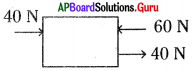The body moves with
A) 20 N; →
B) 60 N; →
C) 20 N; ←
D) 60 N; ←
A) 20 N; →

Question 98.
Stretching of a rubber band with two hands, exerts
A) different magnitudes of forces with opposite directions.
B) Same magnitude of forces with same directions.
C) Different magnitude of forces with same directions.
D) Same magnitude of forces with opposite directions.
A) different magnitudes of forces with opposite directions.Question 99.
The car is moving in the direction of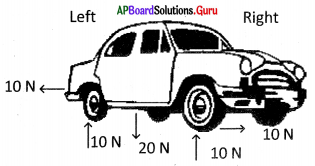A) Left
B) Right
C) Up
D) None
D) None

Question 100.
A) Less surface area
B) More surface area
C) Less surface area of contact
D) More surface area of contact
C) Less surface area of contact

Question 101.
Assertion (A): A boy pushes the tyre again and again with a stick to increase its speed.
If the net force acts in the direction of motion, the speed of an object moving with constant speed also increase.
A) A and R are true, R supports A
B) A and R are true, R doesn’t support A
C) A and R are false
D) A is true and R is false
A) A and R are true, R supports A

Question 102.
Needles has a sharp tip, because
A) Effective pressure is more due to smaller area of contact
B) Effective pressure is less due to smaller area of contact
C) Effective pressure is more due to larger area of contact
D) Effective pressure is less due to larger area of contact
A) Effective pressure is more due to smaller area of contactQuestion 103.
Comb your dry hair, bring the comb near small pieces of paper. Force you observed is
A) magnetic force
B) electrostatic force
C) gravitational force
D) normal force